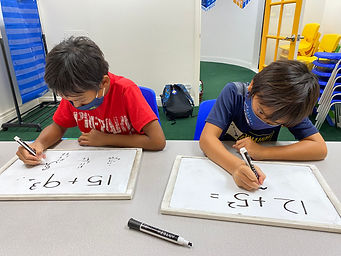## Ms. Amanda

### Target 1​

###### Lesson Type:

Continuation

Number Operation

:

Computation

Evaluate arithmetic expressions that include an exponent.

###### 1:

Understand that an exponent indicates the number of times that a base is multiplied by itself.

###### 2:

Write a repeating multiplication equation that is equivalent to a given exponent.

6th

###### Vocabulary:

Exponent, Multiply

Activities:

• Students were given an exponent and wrote multiplication number sentences to match the exponent.
• Students solved number sentences involving exponents.### Home Exploration

###### Guiding Questions:## Absent Students:

### Target 2

:

###### 1:

Identify the unknown information in problems.

6th

###### Vocabulary:

Variable

Activities:

• Students solved equations to find the unknown number.### Home Exploration

###### Guiding Questions:### Target 3

:

###### 1:

Define an equal sign as a symbol which shows that the quantity on both sides are the same.

###### 2:

Understand that an equal sign can link an equation to its solution or that it can link two equations together.

6th

###### Vocabulary:

Equivalent

Activities:

• Students found the quantity of one side of an equation and made the other side of the equation equal.### Home Exploration